##全国校区# 吊炸天！十行代码我把情书藏进了小姐姐的微信头像里2021-09-14

# 模块安装

`pip install Pillow`

# 初步使用

1、新建图片并保存

```from PIL import Image

img = Image.new("RGB", (512, 512), (0, 100, 200)) # 创建一张新的图片 Image.new(mode, size, color=0)  img.save("img.png")```2、重新绘制图片

```from PIL import Image

img = Image.open("img.png") # 打开图片 draw = ImageDraw.Draw(img) # 取得绘图对象，用于绘制对角线 draw.line((0, 0, img.size, img.size), fill=128, width=2)
draw.line((0, img.size, img.size, 0), fill=128, width=2)
img.save("img-line.png")```3、图片合并

```from PIL import Image

img = Image.open("img.png") # 打开图片 img_small = Image.new("RGB", (32, 32), 'red') # 创建图片 img.paste(img_small, (20, 20)) # 将 img_small 粘贴到 img 的 (20,20) 位置处 img.save("img-paste.png")```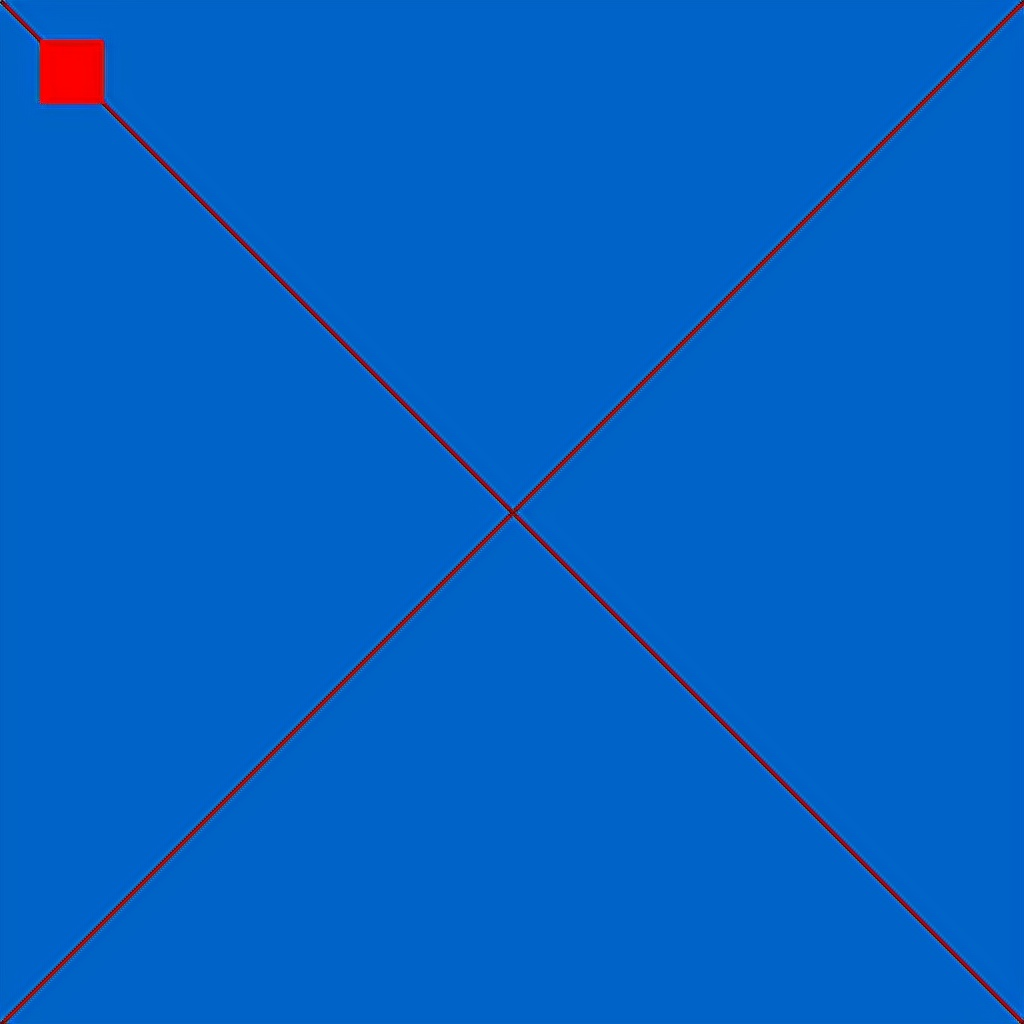# 放大招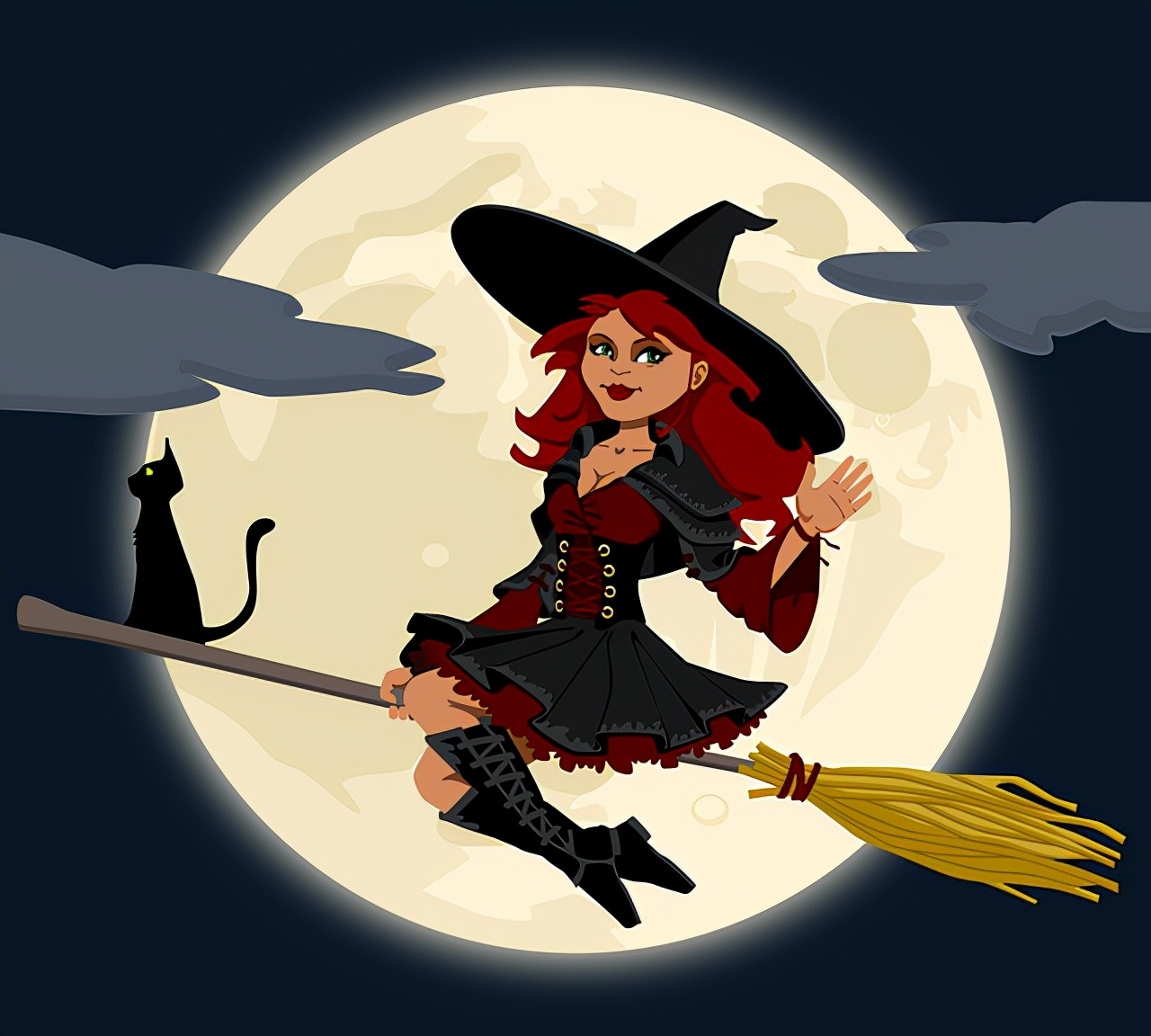```from PIL import Image, ImageDraw, ImageFont

img_child_size = 15 text = "今晚的月色真美" font = ImageFont.truetype('AliPuHui-Bold.ttf', img_child_size)
img_path = './moon.png' ```

```img = Image.open(img_path)
img_w, img_h = img.size
img_child = Image.new("RGB", (img_child_size, img_child_size))
img_ans = Image.new("RGB", (img_child_size * img_w, img_child_size * img_h))```

`text_w, text_h = font.getsize("中") # 获取单个文字的宽、高 offset_x = (img_child_size - text_w) >> 1 # 文字水平居中 offset_y = (img_child_size - text_h) >> 1 # 文字垂直居中 char_index = 0 draw = ImageDraw.Draw(img_child) # 小图的绘图对象，用于绘制文字 for x in range(img_w): # 宽在外 高在内，因此文字的方向是从左到右，从上到下排列的 for y in range(img_h): draw.rectangle((0, 0, img_child_size, img_child_size), fill='lightgray') # 绘制背景，看起来会好一些 draw.text((offset_x, offset_y), text[char_index], font=font, fill=img.getpixel((x, y))) # 用（x,y）处像素点的色值绘制字体 img_ans.paste(img_child, (x * img_child_size, y * img_child_size)) char_index = (char_index + 1) % len(text) img_ans.save('moon-text.png') `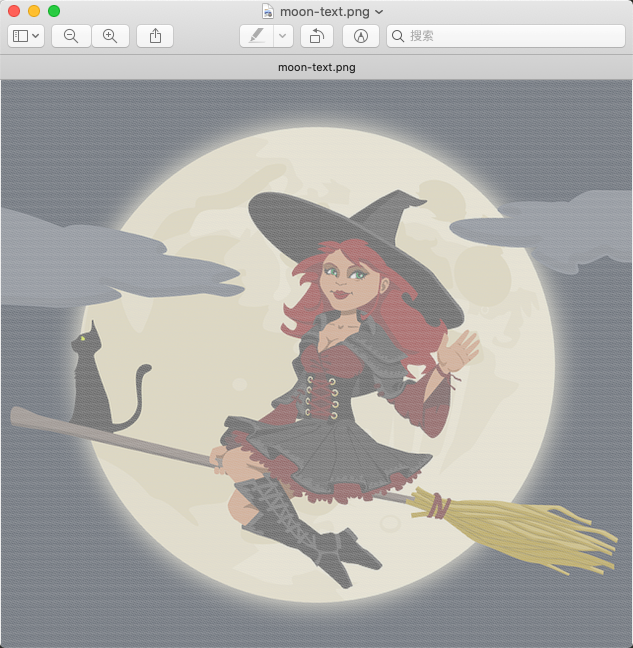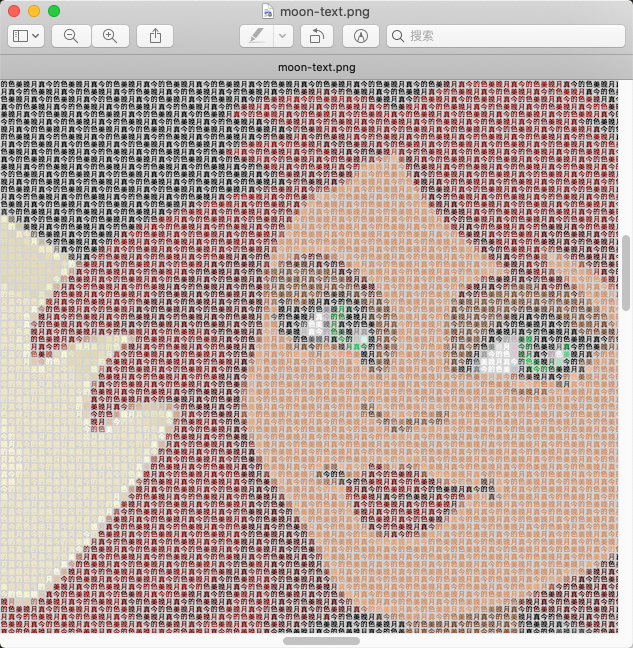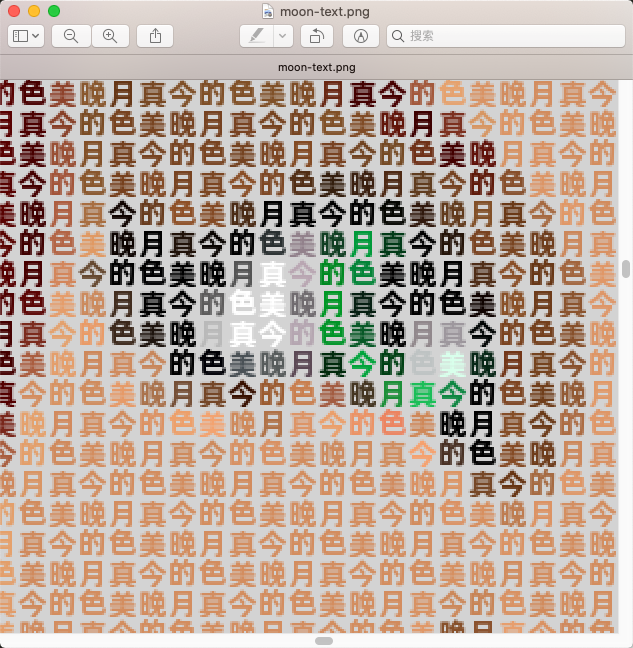OK